• 大三上学期做得编译原理实验，这是最后一个，是把词法分析器、语法分析器、解释器运行全都和在一块，最后运行可以得到相应的结果。
• 编译原理\编译原理课程讲义\编译原理实验三，四讲稿编译原理\编译原理课程讲义\编译原理实验三，四讲稿编译原理\编译原理课程讲义\编译原理实验三，四讲稿
• 合工大 编译原理 实验三 LR(1) 分析法 本项目使用c++实现，利用Windows API制作了简易的UI界面。 具体功能如下： 支持查看文法，项目族，LR(1) 分析表，句子归约过程。 可使用包含左递归的文法且在过程中不生成新...
合工大 编译原理 实验三 LR(1) 分析法
本项目使用c++实现，利用Windows API制作了简易的UI界面。
具体功能如下：

支持查看文法，项目族，LR(1) 分析表，句子归约过程。
可使用包含左递归的文法且在过程中不生成新终结符
利用Graphviz查看DFA转换图

详细功能、示例截图、项目代码请见GitHub：LR(1)分析
部分核心代码如下：
1.求FIRST集（该方法尚属测试阶段，如有问题欢迎大佬批评指出）
//利用检测FIRSTset变动情况来求FIRST集的函数，试图忽视左递归与回溯，并不产生新的非终结符
void CheckLR1class::getFIRSTsets() {
multimap<string, string> CopyedGrammarFormula = GrammarFormula;		//备份的文法表达式列表

while (true) {
bool isFIRSTsetincreased = false;//本次遍历是否存在FIRST集变动

for (auto i = FIRSTset.begin(); i != FIRSTset.end() && isFIRSTsetincreased == false; i++) {		//删去first集中存在空字符且在产生式右部首位的字符，这样可以保证遍历到B->Ab,A->€中的b
if (i->second.find(NULLCHARACTER) != i->second.end()) {
for (auto ii = CopyedGrammarFormula.begin(); ii != CopyedGrammarFormula.end(); ii++) {
if (ii->first.at(0) == i->first) {

auto oldii = ii;
CopyedGrammarFormula.insert(make_pair(ii->first.substr(1),ii->second));
ii++;
CopyedGrammarFormula.erase(oldii);

isFIRSTsetincreased = true;

}
}
}
}

for (auto i = CopyedGrammarFormula.begin(); i != CopyedGrammarFormula.end(); i++) {
//ReversedGrammarFormula.insert(i->second, i->first);
if (Vnset.find(i->first.at(0)) == Vnset.end() && (FIRSTset.find(i->second.at(0))== FIRSTset.end() || FIRSTset[i->second.at(0)].find(i->first.at(0)) == FIRSTset[i->second.at(0)].end())) {	//终结符且未收录直接添加
FIRSTset[i->second.at(0)].insert(i->first.at(0));
isFIRSTsetincreased = true;
}
if (Vnset.find(i->first.at(0)) != Vnset.end() && FIRSTset[i->first.at(0)].size() != 0) {						//非终结符且该符号非终结符非空
bool isallhasincluded = true;																					//若存在不包括则添加

for (auto tmpj = FIRSTset[i->first.at(0)].begin(); tmpj != FIRSTset[i->first.at(0)].end(); tmpj++) {//判断右侧非终结符FIRST集是否已被包含在自身FIRST集中，如果包含则不添加，并不视为FIRST集改动；否则添加，并记录为FIRST集改动
bool isthishasincluded = false;
for (auto tmpi = FIRSTset[i->second.at(0)].begin(); tmpi != FIRSTset[i->second.at(0)].end(); tmpi++) {
if (*tmpi == *tmpj && *tmpj!=NULLCHARACTER) {
isthishasincluded = true;
}
}
if (isthishasincluded == false) {
isallhasincluded = false;
}
}

if (isallhasincluded == false) {
for (auto tmpj = FIRSTset[i->first.at(0)].begin(); tmpj != FIRSTset[i->first.at(0)].end(); tmpj++) {
if (*tmpj != NULLCHARACTER) {
FIRSTset[i->second.at(0)].insert(*tmpj);
isFIRSTsetincreased = true;
}
}
}
}
}

if (isFIRSTsetincreased == false) {
break;
}
}

}

2.制作DFA分析
本函数使用队列来进行生成DFA，队列中储存需要展开（求取其子节点）的项目族，每次取队列中的队首进行展开，并将其子节点添加到队列中（前提为未曾在队列中出现过）。
void CheckLR1class::makeAnalysedSheet() {
statusblock initalstatusblock;

/*tmp.GrammarSentence = string{ mostcharacter };
tmp.GrammarVn = "0";
tmp.index = 0;*/
SymbolSet tmpsymbolset;
tmpsymbolset.insert('#');
//tmp.SearchSymbol = tmpsymbolset;
ProjectSentence tmp = make_tuple("0", string{ mostcharacter }, tmpsymbolset, 0);
initalstatusblock.ProjectItem.insert(tmp);
initalstatusblock += getCLOSURE(initalstatusblock);

//set<set<ProjectSentence>> alreadyexpanedstatusblock;		//已经展开过的状态族集合的集合 由于statusblock结构中存在自增变量无法利用
stack<statusblock> searchingstack;
searchingstack.push(initalstatusblock);

while (searchingstack.empty() == false) {
statusblock currentstatusblock = searchingstack.top();

collapsesameFormula(currentstatusblock);

searchingstack.pop();
SymbolSet symbol_iter = getSymbols(currentstatusblock);

for (auto i = symbol_iter.begin(); i != symbol_iter.end(); i++) {
statusblock tmpfetchedstatusblock = GO(currentstatusblock, *i);

collapsesameFormula(tmpfetchedstatusblock);

GOset[currentstatusblock][*i] = tmpfetchedstatusblock;

bool isfinded = false;

for (auto GOset_iter = GOset.begin(); GOset_iter != GOset.end(); GOset_iter++) {
if (GOset_iter->first == tmpfetchedstatusblock) {
isfinded = true;
}
}

if(isfinded==false )		//尚未进行展开
searchingstack.push(tmpfetchedstatusblock);
}
}
//该函数产生父节点与子节点之间的对应关系map，故后续需转化输出处理
}



展开全文• 编译原理实验三：正规文法到正规式的转换,zip文件里包含实验报告和源代码两部分。
• 这是哈工大编译原理实验语义分析的实验指导书，自我感觉还是不错的
• 湖南大学专业课编译原理实验的相关资料，实验分巨高，另外推荐陈果老师，讲课真的好，祝大家学业有成，代码以及报告仅作参考，不要过分摘抄。
• 代码链接：编译原理实验三 文章目录实验三 语法分析程序（一）学习经典的语法分析器（1学时）一、实验目的二、实验任务三、实验内容（二）实现一门语言的语法分析器（3学时）一、实验目的二、实验任务三、实验内容...
代码链接：编译原理实验三
文章目录实验三 语法分析程序（一）学习经典的语法分析器（1学时）一、实验目的二、实验任务三、实验内容（二）实现一门语言的语法分析器（3学时）一、实验目的二、实验任务三、实验内容实验步骤学习经典的语法分析器（TINY）实现一门语言的语法分析器（TINY）语言确定BNF文法到EBNF文法的转换抽象语法树结构语法分析器LL(1)语法分析器的实现基础数据存储方式解析产生式NULLABLE集合计算FIRST集合计算FOLLOW集合计算FIRST_S集合计算产生预测分析表parse语法分析生成语法树树结点的声明结点的构造子结点个数的分配回溯得到正确的结点名修改后的语法分析过程实验结果输出结果说明
实验三 语法分析程序
（一）学习经典的语法分析器（1学时）
一、实验目的
学习已有编译器的经典语法分析源程序。
二、实验任务
阅读已有编译器的经典语法分析源程序，并测试语法分析器的输出。
三、实验内容

选择一个编译器，如：TINY，其它编译器也可（需自备源代码）。

阅读语法分析源程序，加上你自己的理解。尤其要求对相关函数与重要变量的作用与功能进行稍微详细的描述。若能加上学习心得则更好。TINY语言请参考《编译原理及实践》第3.7节。对TINY语言要特别注意抽象语法树的定义与应用。

测试语法分析器。对TINY语言要求输出测试程序的字符形式的抽象语法树。（手工或编程）画出图形形式的抽象语法树。

TINY语言：
测试用例一：sample.tny。
（二）实现一门语言的语法分析器（3学时）
一、实验目的
通过本次实验，加深对语法分析的理解，学会编制语法分析器。
二、实验任务
用C或C++语言编写一门语言的语法分析器。
三、实验内容

语言确定：C－语言，其定义在《编译原理及实践》附录A中。也可选择其它语言，不过要有该语言的详细定义（可仿照C－语言）。一旦选定，不能更改，因为要在以后继续实现编译器的其它部分。鼓励自己定义一门语言。也可选择TINY语言，但需要使用与TINY现有语法分析代码不同的分析算法实现，并在实验报告中写清原理。

完成C－语言的BNF文法到EBNF文法的转换。通过这一转换，消除左递归，提取左公因子，将文法改写为LL（1）文法，以适用于自顶向下的语法分析。规划需要将哪些非终结符写成递归下降函数。

为每一个将要写成递归下降函数的非终结符，如：变量声明、函数声明、语句序列、语句、表达式等，定义其抽象语法子树的形式结构，然后定义C－语言的语法树的数据结构。

仿照前面学习的语法分析器，编写选定语言的语法分析器。可以自行选择使用递归下降、LL(1)、LR(0)、SLR、LR(1)中的任意一种方法实现。

准备2~3个测试用例，测试并解释程序的运行结果。

实验步骤
学习经典的语法分析器（TINY）
主要的程序部分位于parse.c文件内
语法树节点的结构：globals.h内
#define MAXCHILDREN 3

typedef struct treeNode {
struct treeNode *child[MAXCHILDREN];
struct treeNode *sibling;
int lineno;
NodeKind nodekind;
union {
StmtKind stmt;
ExpKind exp;
} kind;
union {
TokenType op;
int val;
char *name;
} attr;
ExpType type; /* for type checking of exps */
} TreeNode;

其中重要的部分即child结点，sibling结点以及attr标识参数的结点。在表达式exp部分，op会被赋值为token；在赋值、READ、读取ID部分会对name赋值；在NUM结点则给val赋值。
由于这个parse使用的是递归下降，所以需要一个程序入口来进入，这部分就是TreeNode* parse(void)函数。
具体的话，就如递归下降分析算法，把每一个非终结符看作一个函数，然后调用它，直到到达一个终结符。非终结符构成的函数如：stmt_sequence()，statement()，if_stmt()，repeat_stmt()，assign_stmt()，read_stmt()，write_stmt()，exp()，simple-exp()，term()，factor()，在进入这些函数后，优惠根据产生时进行选择，如果是非终结符，继续调用，否则若是终结符，则需要调用封装的match()函数，判断token是否与之匹配，匹配则继续进行token的接受，否则语法分析失败。
就这样，在递归调用的过程中，到达终结符节点时则进行树结点的建立，从而完成语法树的建立过程。
对输入文件sample.tny生成的语法树
TINY COMPILATION: sample.tny

Syntax tree:
Read: x
If
Op: <
Const: 0
Id: x
Assign to: fact
Const: 1
Repeat
Assign to: fact
Op: *
Id: fact
Id: x
Assign to: x
Op: -
Id: x
Const: 1
Op: =
Id: x
Const: 0
Write
Id: fact

图形形式：实现一门语言的语法分析器（TINY）
语言确定
使用TINY语言，与实验一相同
BNF文法到EBNF文法的转换
BNF文法：
program -> stmt-sequence EOF
stmt-sequence -> stmt-sequence ; statement
stmt-sequence -> statement
statement -> if-stmt
statement -> repeat-stmt
statement -> assign-stmt
statement -> read-stmt
statement -> write-stmt
if-stmt -> if exp then stmt-sequence else stmt-sequence end
if-stmt -> if exp then stmt-sequence end
repeat-stmt -> repeat stmt-sequence until exp
assign-stmt -> identifier := exp
read-stmt -> read identifier
write-stmt -> write exp
exp -> simple-exp comparison-op simple-exp
exp -> simple-exp
comparison-op -> <
comparison-op -> =
simple-exp -> simple-exp addop term
simple-exp' -> term
addop -> +
addop -> -
term -> term mulop factor
term -> factor
mulop -> *
mulop -> /
factor -> ( exp )
factor -> number
factor -> identifier

消除左递归：line 2，line 19，line 23
提取左公因子：line 9
EBNF文法：
program -> stmt-sequence EOF
stmt-sequence -> statement stmt'
stmt' -> ; statement stmt'
stmt' -> $statement -> if-stmt statement -> repeat-stmt statement -> assign-stmt statement -> read-stmt statement -> write-stmt if-stmt -> if exp then stmt-sequence else-part' else-part' -> else stmt-sequence end else-part' -> end repeat-stmt -> repeat stmt-sequence until exp assign-stmt -> identifier := exp read-stmt -> read identifier write-stmt -> write exp exp -> simple-exp cmp-exp' cmp-exp' -> comparison-op simple-exp cmp-exp' ->$
comparison-op -> <
comparison-op -> =
simple-exp -> term simple-exp'
simple-exp' -> addop term simple-exp'
simple-exp' -> $addop -> + addop -> - term -> factor term' term' -> mulop factor term' term' ->$
mulop -> *
mulop -> /
factor -> ( exp )
factor -> number
factor -> identifier

抽象语法树结构
语法树节点与 parse.c 中定义相同，只是每个结点都具有name，且有最大子结点个数和当前访问子结点的编号：
typedef struct treeNode {
struct treeNode *child[MAXCHILDREN];
struct treeNode *father;
int child_no;		// 当前访问的子结点编号
int max_child;		// 最大子结点个数
int lineno;
std::string name;	// 名称
NodeKind nodekind;
union {
StmtKind stmt;
ExpKind exp;
} kind;
union {
TokenType op;
int val;
} attr;
ExpType type; /* for type checking of exps */

} TreeNode;

语法分析器
由于选择了TINY语言，不能使用递归下降算法，所以这里选择LL(1)语法分析器来进行实现
LL(1)语法分析器的实现
构成LL(1)语法分析器，需要求得四个关键集合：NULLABLE集，FIRST集，FOLLOW集，FIRST_S集。得到四个集合后，便可以开始构建LL(1)预测分析表，从而完成准备过程。
完成LL(1)预测分析表之后，就可以对输入程序进行语法分析，通过预测分析表来指导产生式的选择。
基础数据存储方式

产生式：

vector<string> prod_;
unordered_map<int, vector<string>> prod;

prod_以字符串形式存储每一条未被解析过的产生式，具体格式即上述的EBNF语法的产生式。
prod则是将每条产生式解析后作为val，key为该条产生式所在的行数，如上述样例中
line 13: repeat-stmt -> repeat stmt-sequence until exp

得到的prod的key为13，val则为:
[repeat-stmt, repeat, stmt-sequence, until, exp]

将每条产生式读入后如此解析，放入prod中

终结符与非终结符：

set<string> nonterminal;
vector<TokenType> terminal;

可以都看作是数组的形式存储，不过在nonterminal中，为了方便之后的查找使用了set进行存储，提高查找的效率。

NULLABLE，FIRST，FOLLOW，FIRST_S集

set<string> nullable;
unordered_map<string, set<TokenType>> first;
unordered_map<string, set<TokenType>> follow;
unordered_map<int, set<TokenType>> first_s;

对于NULLABLE来说，只需要知道某个非终结符是不是NULLABLE即可，所以使用set存储；而FIRST和FOLLOW则是对于每一个非终结符，都要维持一个终结符构成的集合，所以使用hashmap嵌套set实现；而FIRST_S集则是对于每一个产生式都有一个终结符集合，依旧是通过产生式的编号作为键值。

预测分析表

unordered_map<string, unordered_map<TokenType, vector<int>>> LL1_table;

预测分析表中，由于对于每一个非终结符，对其来说每一个终结符都要有一个相应的转移，但是转移的产生式不唯一，所以使用vector来进行保存，产生式依旧采用编号来进行识别。
解析产生式
目的：由于最初输入的是一个字符串形式的产生式，存放在prod_内，但最终为了方便使用，需要将每一个终结符和非终结符解析存储在prod内。
算法：对于产生式头部和产生式体，通过"->“就可以分离。对于产生式体的分离，则每次找到” "所在的位置，以此来进行分割，直到没有空格存在。
具体实现：
vector<string> parse(const string &production) {
vector<string> ans; // 存放最终的字符串
int i;
int before = 0;
for (i = 0; i < production.length(); i++) {
if (production[i] == '-' && production[i + 1] == '>') { // 找到产生式头部的分割点
string head = production.substr(0, i - 1);
ans.push_back(head);
i += 3; // 指针移至产生式体的第一个字符
before = i;
break;
}
}
for (; i < production.length(); i++) {
if (production[i] == ' ') {	// 找空格
string nxt = production.substr(before, i - before); // 分割
ans.push_back(nxt);
before = i + 1;
}
}
string nxt = production.substr(before, i - before);
ans.push_back(nxt);
return ans;
}

NULLABLE集合计算
这一部分根据产生式来看，只有能直接推出空串的产生式可以在NULLABLE中
具体实现：
void get_nullable() {
for (auto s : prod_) {
int end;
for (int i = 0; i < s.length(); i++) {
if (s[i] == '-' && s[i + 1] == '>')
end = i - 1;
if (s[i] == '$') { // 如果能直接推出空串 string ret = s.substr(0, end); nullable.insert(ret); // 属于NULLABLE集 } } } return; }  FIRST集合计算 这一部分根据上课所讲的伪代码进行实现即可如果产生式体第一个为终结符，那么其FIRST集即为该终结符；若为非终结符，则该FIRST集应并上该非终结符的FIRST集，若其属于NULLABLE集，则继续向后看，不属于那么直接退出。 直到没有一个FIRST集再被修改过，就表示求解完成。 具体实现：  void get_First() { int flag = 1; // 判断是否还有FIRST集改变 while (flag) { flag = 0; for (int i = 0; i < prod_num; i++) { // 遍历所有产生式 string head = prod[i]; set<TokenType> origin = first[head]; int size = origin.size(); for (int j = 1; j < prod[i].size(); j++) { // 遍历该产生式体 if (prod[i][j] == "$") // 空集跳过
continue;
string nxt = prod[i][j];
if (nonterminal.find(nxt) != nonterminal.end()) { // 是非终结符
set<TokenType> temp = first[nxt];
set_union( // 取并集
origin.begin(), origin.end(), temp.begin(), temp.end(),
inserter(origin, origin.begin()));
if (nullable.find(nxt) == nullable.end()) { // 不在NULLABLE，退出内循环
break;
}
} else { // 是终结符，加入后退出内循环
TokenType token = get_Terminal(nxt, head);
origin.insert(token);
break;
}
}
if (origin.size() != size) // 当前FIRST集被修改，while大循环继续执行
flag = 1;
first[head] = origin;
}
}
}

FOLLOW集合计算
伪代码：与FIRST集不同的，是其对产生式体的每一个非终结符进行，并且是逆序执行。
首先设置temp集合暂存可能赋值的FOLLOW，逆序遍历，如果是终结符，则temp集合为该终结符；如果是非终结符，则该非终结符的FOLLOW与temp取并集，并判断是否在NULLABLE中，不在NULLABLE中则temp修改为其FIRST集，否则并其FIRST集。
具体实现：
    void get_Follow() {
int flag = 1; // 判断是否还有FOLLOW集改变
while (flag) {
flag = 0;
for (int i = 0; i < prod_num; i++) { // 对每条产生式遍历
string head = prod[i];
set<TokenType> temp = follow[head];

for (int j = prod[i].size() - 1; j >= 1; j--) { // 逆序
if (prod[i][j] == "$") // 空跳过 continue; string nxt = prod[i][j]; if (nonterminal.find(nxt) != nonterminal.end()) { // 是非终结符 set<TokenType> upd = follow[nxt]; int size = upd.size(); set_union( temp.begin(), temp.end(), upd.begin(), upd.end(), inserter(upd, upd.begin())); // 取并集 if (upd.size() != size) { // FOLLOW集被修改，while大循环继续 flag = 1; } follow[nxt] = upd; // 更新当前非终结符的FOLLOW if (nullable.find(nxt) == nullable.end()) { // 不在NULLABLE中 temp.clear(); // 修改temp temp = first[nxt]; } else { // 在NULLABLE中 set<TokenType> ret = first[nxt]; set_union( // 取并集 temp.begin(), temp.end(), ret.begin(), ret.end(), inserter(temp, temp.begin())); } } else { // 是终结符 TokenType token = get_Terminal(nxt, head); temp.clear(); // temp清空，修改为当前的终结符 temp.insert(token); } } } } }  FIRST_S集合计算 求解FIRST_S集要依赖于FIRST，FOLLOW，NULLABLE集。 FIRST_S集的求法与FIRST相似，只不过是对每个产生式进行，与FIRST不同的，如果遍历到了最后一个符号，那么需要该产生式的FIRST集并上产生式头的FOLLOW集具体实现：  void cal_First_s(int i) { string head = prod[i]; // 以下部分与FIRST相似 set<TokenType> origin = first_s[i]; for (int j = 1; j < prod[i].size(); j++) { string nxt = prod[i][j]; if (nxt == "$")
continue;
if (nonterminal.find(nxt) != nonterminal.end()) {
set<TokenType> temp = first[nxt];
set_union(
temp.begin(), temp.end(), origin.begin(), origin.end(),
inserter(origin, origin.begin()));
first_s[i] = origin;
if (nullable.find(nxt) == nullable.end()) {
return;
}
} else {
TokenType token = get_Terminal(nxt, head);
origin.insert(token);
first_s[i] = origin;
return;
}
}
set<TokenType> fl = follow[head];
set_union( // 遍历到最后一个，取其FOLLOW并集
fl.begin(), fl.end(), origin.begin(), origin.end(), inserter(origin, origin.begin()));
first_s[i] = origin; // 修改当集FIRST_S
}
void get_First_s() {
for (int i = 0; i < prod_num; i++) { // 对每条产生式处理
cal_First_s(i);
}
}

产生预测分析表
求完上述的集合后，就可以根据FIRST_S集求得具体的LL(1)预测分析表。
当上述集合求完后，很显然，最终集合中都保存的为终结符。构造预测分析表的算法极其简单，对于每一条语句的产生式头部，他在当前语句的FIRST_S集中的终结符的转一下，会得到该条语句，具体实现如下：
    void get_Transtable() {
for (auto it : first_s) { // 对每一个FIRST_S集
int idx = it.first; // 获得该产生式的编号
string head = prod[idx]; // 得到对应的产生式头部
for (auto trans : it.second) { // 遍历FIRST_S集
LL1_table[head][trans].push_back(idx); // 在该token下转移到该产生式
}
}
}

parse语法分析
在有了预测分析表之后，就可以结合词法分析器进行语法分析。
伪代码：先压入一个起始符号，生成具体的产生式，当栈不为空时，判断栈顶元素，栈顶元素为非终结符，则将其弹出，根据当前词法分析器获得的token在预测分析表中获得对应的产生式，将产生式体从右向左压入栈中，如果没有对应的产生式，那么是错误情况；如果是终结符，token与其相匹配的话，那么弹出，获得新token，否则就是错误情况。
具体实现：
    stk.push("program"); // 其实符号
token = ERROR;
token = getToken(fp); // 得到第一个token
while (!stk.empty()) {
print_stack_info(stk); // 打印栈信息
string top = stk.top(); // 取栈顶
stk.pop();	// 弹出
if (nonterminal.find(top) == nonterminal.end()) { // 终结符
TokenType tt = ll1.get_Terminal(top); // 得到这个终结符的Token表时
if (tt == token) { // 匹配，则获得下一个token
token = getToken(fp);
} else { // 触发ERROR函数，打印错误信息
ERROR_FUNC(head, "", tt);
}
} else { // 非终结符
vector<int> nxt_prod = ll1.LL1_table[top][token]; // 从预测分析表取出产生式
if (nxt_prod.size() == 0) { // ERROR！
ERROR_FUNC(head, top);
} else {
vector<string> ret = ll1.prod[nxt_prod]; // 取出产生式体
for (int i = ret.size() - 1; i >= 1; i--) { // 反向压栈
if (ret[i] == "$") // 空串不压栈 continue; stk.push(ret[i]); } } } }  经过上述过程即可完成语法分析，正确则会打印完整的栈信息，并且输出YES 否则输出相应的错误信息 生成语法树 除了做语法分析之外，还要生成相关的中间代码：抽象语法树 考虑到上述语法分析过程是先压栈，根据栈顶弹出再压栈的过程，此时考虑树的前序遍历算法，如果想要保存每一步遍历的信息，其过程是：压入根节点，弹出栈顶元素，生成左子结点，右子结点，右子结点入栈，左子结点入栈，重复进行，直到到达根节点，只需要出栈。那么这就比较显然了：上述的语法分析过程即树的前序遍历。从而，只需要将前序遍历算法反向，就可以构造出一颗树，也就是上述的语法分析过程即反向构建树的过程。 有了上述的算法基础，就可以着手开始构造树了。 树结点的声明 (参考tiny_tm/globals.h)： typedef struct treeNode { struct treeNode *child[MAXCHILDREN]; // 子结点 struct treeNode *father; // 指向父结点，方便回溯 int child_no; // 当前遍历的子结点编号（构造树时有用） int max_child; // 最大的子结点编号 int lineno; std::string name; // 当前结点的名称 NodeKind nodekind; union { StmtKind stmt; ExpKind exp; } kind; union { TokenType op; int val; } attr; ExpType type; /* for type checking of exps */ } TreeNode;  结点的构造 构造树的根结点： TreeNode *newRootNode(string name) { TreeNode *t = new TreeNode; // new一个，然后相应的初始化即可 t->lineno = line_no; t->child_no = 0; t->max_child = -1; // 这里设置为-1为了方便后续的判断，关于子结点数目的处理见后续函数 t->nodekind = StmtK; t->name = name; return t; }  普通结点： TreeNode *newNode(TreeNode *fa, string name) { TreeNode *t = new TreeNode; set<string> nonterminal = ll1.get_nonterminal(); if (nonterminal.find(name) != nonterminal.end()) { t = newStmtNode(name); // 如果是非终结符，则调用上述函数 } else { t->lineno = line_no; t->child_no = 0; t->max_child = 0; // 终结符结点没有子结点，在后续也不需要resize t->nodekind = ExpK; TokenType tt = ll1.get_Terminal(name); // 得到当前非终结符的名称 t->attr.op = tt; t->name = name; } t->father = fa; // 设置父节点 return t; }  有了上述的函数，就可以构造出理想中的数据结构，初始时初始化根节点：  // 生成根节点 TreeNode *t = new TreeNode; t = newRootNode("program"); // 根节点指针，用于发生错误时输出语法树 TreeNode *head = t;  对于其他节点的生成，由于压栈的过程是在非终结符时进行，所以压栈时，反向建立当前结点的子结点：  t->child[i - 1] = newNode(t, ret[i]);  子结点个数的分配 在生成子结点时，值得注意的是，当前结点应当分配最大子结点个数，所以在弹栈时，对当前结点resize： 为什么不在上面生成子结点时就设置子节点的最大个数呢，因为当时没有得到对应的token，只有得到对应阶段的token才能进行子结点个数的确定。  string top = stk.top(); stk.pop(); resize_treenode(t, head);  resize_treenode函数： // 重新分配当前结点的最大子结点个数 // 因为在生成子结点时, 没有对应的token, 无法得到正确的最大子结点个数 void resize_treenode(TreeNode *t, TreeNode *head) { t->child_no = 0; // 初始子结点编号为0 if (t->nodekind == ExpK) t->max_child = 0; else { vector<int> nxt_prod = ll1.LL1_table[t->name][token]; // 得到相应的产生式 if (nxt_prod.size() == 0) { ERROR_FUNC(head, t->name, token); } if (ll1.prod[nxt_prod] == "$")
t->max_child = 0;
else
t->max_child = ll1.prod[nxt_prod].size() - 1; // 设置最大的子节点个数
}
}

回溯
由于到达叶子节点即终结符，需要进行回溯，到达非终结符结点时，如果其子结点都被遍历，也许要回溯，此时要回溯到最近的没有完全访问的父结点的第一个未被访问子结点。
对于叶子节点来说，其父结点一定是非终结符结点，只需要指向其父结点，并且当子结点未被全访问时，指向其子结点，否则交给后续非终结符结点的回溯过程进行；对非终结符结点，当他的子结点全被访问过，或者最大子结点为-1（还未分配）时，需要回溯，而如果到达了叶子节点或者根节点，此时即可停止回溯，具体回溯的过程，即指向父结点，并找到第一个未被访问的子结点，而如果这一个子结点还未被分配，那么就应当结束循环。
这一个回溯过程还包括对于新生成的子结点的访问。
具体实现:
// 回溯, 找到最近的一个还有未被访问过的子结点的结点
TreeNode *get_Free_Node(TreeNode *t, int pos) {
if (pos == 1) { // 如果是新生成了子结点，则特判，并且指向其子结点
if (t->max_child != 0) {
return t->child[t->child_no++];
}
}
if (t->nodekind == ExpK && t->father != NULL) { // 叶子节点
t = t->father;
if (t->child_no < t->max_child) {
t = t->child[t->child_no++];
}
}
if (t->nodekind == StmtK) { // 非终结符结点
while (t->child_no >= t->max_child && t->max_child != -1 && t->nodekind == StmtK &&
t->father != NULL) {
t = t->father;
if (t->child_no < t->max_child) { // 指向第一个为访问的结点
t = t->child[t->child_no++];
if (t->max_child == -1) { // 得到一个未被分配的结点
break;
}
}
}
}
return t;
}

得到正确的结点名
由于在初始化结点时，并没有办法得到一个正确的token，所以得到的名称也是不正确的，所以需要在匹配终结符时进行重命名，只需要赋值词法分析中的currString即可实现：
// 对结点进行重命名, 以显示当前ID或NUM接收到的具体的字符串
void rename(TreeNode *t) {
t->name = currString;
}

修改后的语法分析过程
    stk.push("program");
token = ERROR;
token = getToken(fp);

// 生成根节点
TreeNode *t = new TreeNode;
t = newRootNode("program");
// 根节点指针，用于发生错误时输出语法树
TreeNode *head = t;

while (!stk.empty()) {
print_stack_info(stk);
string top = stk.top();
stk.pop();

resize_treenode(t, head); // 重分配

if (nonterminal.find(top) == nonterminal.end()) {
TokenType tt = ll1.get_Terminal(top);
if (tt == token) {
rename(t); // 重命名
token = getToken(fp);
// 语法树回溯, 因为到达了叶子节点
t = get_Free_Node(t, 0);
} else { // ERROR!
ERROR_FUNC(head, "", tt);
}
} else {
vector<int> nxt_prod = ll1.LL1_table[top][token];
if (nxt_prod.size() == 0) { // ERROR！
ERROR_FUNC(head, top);
} else {
vector<string> ret = ll1.prod[nxt_prod];
for (int i = ret.size() - 1; i >= 1; i--) {
if (ret[i] == "$") // 空串不压栈 continue; stk.push(ret[i]); // 生成当前结点子结点，但不分配最大子结点大小 t->child[i - 1] = newNode(t, ret[i]); } } // 看当前非叶子结点是否需要回溯：可能所以子结点都被遍历 // 若不需要，则进入下一个子结点继续 t = get_Free_Node(t, 1); } }  实验结果 具体结果说明见后文或README.md 运行正例即pos.tny { Sample program in TINY language - computes factorial } read x; if 0 < x then { don't compute if x <= 0 } fact := 1; repeat fact := fact * (x / 3); x := x - 1 + 0 until x = 0; write fact { output factorial of x } else write fact end  输出的栈： program --------------- Token = reserved word: read stmt-sequence EOF --------------- Token = reserved word: read …… read identifier stmt' EOF --------------- Token = reserved word: read identifier stmt' EOF --------------- Token = ID, name= x stmt' EOF --------------- Token = ; …… YES  得到的语法树（部分）： |program |stmt-sequence |statement |read-stmt |reserved word: read |ID, name= x |stmt' |; |statement |if-stmt |reserved word: if |exp |simple-exp |term |factor |NUM, val= 0 |term' |simple-exp' |cmp-exp' |comparison-op |< |simple-exp |term |factor |ID, name= x |term' |simple-exp' |reserved word: then |stmt-sequence  运行反例neg.tny { Sample program in TINY language - computes factorial } read x; else 0 < x then { don't compute if x <= 0 } fact := 1; repeat fact := fact * (x / 3); x := x - 1 + 0 until x = 0; write fact { output factorial of x } else write fact end  输出栈： ; statement stmt' EOF --------------- Token = ; statement stmt' EOF --------------- Token = reserved word: else ERROR! now token: 4 nonterminal: statement top token: reserved word: else  输出语法分析树： |program |stmt-sequence |statement |read-stmt |reserved word: read |ID, name= x |stmt' |; |statement |stmt' |EOF  与预期相符。 输出结果说明 read identifier stmt' EOF --------------- Token = reserved word: read  上方为stack的信息，顶行为栈顶；下方则是当前得到的token 在文件的末尾，如果输出YES，表示语法正确，否则会输出相关错误信息。 语法树保存在 output/SyntaxTree 内，不过具体的格式也没有很好看的形式，大概懂什么意思即可。每一列输出的为同一层，在该列的后面且该行的下面为其子结点，如： |program |stmt-sequence |statement |read-stmt |reserved word: read |ID, name= \ |stmt'  statement和stmt’是sibling结点，位于同一层，都是stmt-sequence的子结点；read-stmt是statement的子结点但不是stmt’的子结点 关于代码如何使用，请见README.md  展开全文• 哈工大软件学院编译原理三实验，最后一次实现了一半，通过检查还是可以的，包括代码和文档 • 实验三 语法分析程序 （一）学习经典的语法分析器 一、实验目的 学习已有编译器的经典语法分析源程序。 二、实验任务 阅读已有编译器的经典语法分析源程序，并测试语法分析器的输出。 三、实验内容 （1）选择一个... 实验要求 代码已开源：https://github.com/LinXiaoDe/Quary/tree/master/lab3 实验三 语法分析程序 （一）学习经典的语法分析器 一、实验目的 学习已有编译器的经典语法分析源程序。 二、实验任务 阅读已有编译器的经典语法分析源程序，并测试语法分析器的输出。 三、实验内容 （1）选择一个编译器，如：TINY，其它编译器也可（需自备源代码）。 （2）阅读语法分析源程序，加上你自己的理解。尤其要求对相关函数与重要变量的作用与功能进行稍微详细的描述。若能加上学习心得则更好。TINY语言请参考《编译原理及实践》第3.7节。对TINY语言要特别注意抽象语法树的定义与应用。 （3）测试语法分析器。对TINY语言要求输出测试程序的字符形式的抽象语法树。（手工或编程）画出图形形式的抽象语法树。 TINY语言： 测试用例一：sample.tny。 （二）实现一门语言的语法分析器 一、实验目的 通过本次实验，加深对语法分析的理解，学会编制语法分析器。 二、实验任务 用C或C++语言编写一门语言的语法分析器。 三、实验内容 （1）语言确定：C－语言，其定义在《编译原理及实践》附录A中。也可选择其它语言，不过要有该语言的详细定义（可仿照C－语言）。一旦选定，不能更改，因为要在以后继续实现编译器的其它部分。鼓励自己定义一门语言。也可选择TINY语言，但需要使用与TINY现有语法分析代码不同的分析算法实现，并在实验报告中写清原理。 （2）完成C－语言的BNF文法到EBNF文法的转换。通过这一转换，消除左递归，提取左公因子，将文法改写为LL（1）文法，以适用于自顶向下的语法分析。规划需要将哪些非终结符写成递归下降函数。 （3）为每一个将要写成递归下降函数的非终结符，如：变量声明、函数声明、语句序列、语句、表达式等，定义其抽象语法子树的形式结构，然后定义C－语言的语法树的数据结构。 （4）仿照前面学习的语法分析器，编写选定语言的语法分析器。可以自行选择使用递归下降、LL(0)、LR(0)、SLR、LR(1)中的任意一种方法实现。 （5）准备2~3个测试用例，测试并解释程序的运行结果。 第一部分 学习经典的语法分析器 实验任务： 阅读已有编译器的经典语法分析源程序，并测试语法分析器的输出。 （1）选择一个编译器，如：TINY，其它编译器也可（需自备源代码）。 （2）阅读语法分析源程序，加上你自己的理解。尤其要求对相关函数与重要变量的作用与功能进行稍微详细的描述。若能加上学习心得则更好。TINY语言请参考《编译原理及实践》第3.7节。对TINY语言要特别注意抽象语法树的定义与应用。 （3）测试语法分析器。对TINY语言要求输出测试程序的字符形式的抽象语法树。（手工或编程）画出图形形式的抽象语法树。 TINY语言： 测试用例一：sample.tny。 一. 阅读已有编译器语法分析程序 我选择的经典词法分析程序是TINY源码，下面我就变量作用介绍，函数功能，语法树构建方法以及样例测试这4个方面对TINY词法分析器进行心得总结： 二.变量定义和作用 在头文件globals.h中我们定义了一些节点类型的变量和结构，T I N Y有两种基本的结构类型：语句和表达式。语句共有 5类（ i f语句、 r e p e a t语句、 a s s i g n语句、 r e a d语句和w r i t e语句） ，表达式共有 3类（算符表达式、常量表达式和标识符表达式） 。 因此，语法树节点首先按照它是语句还是表达式来分类，接着根据语句或表达式的种类进行再次分类。树节点最大可有 3个孩子的结构（仅在带有 e l s e部分的i f语句中才需要它们） 语句通过同属域而不是使用子域来排序。 ①节点类型： /*用于分析的语法树*/ typedef enum {StmtK,ExpK} NodeKind; //节点类型  ②表达式类型： typedef enum {IfK,WhileK,ForEach,AssignK,InputK,PrintK} StmtKind; //语句类（statment）  ③表达式种类： typedef enum {OpK,ConstK,IdK} ExpKind; //表达式类（expression）算符表达式、常量表达式和标识符表达式  ④语法树的节点定义： 树的结点种类NodeKind分为StmtK和ExpK两类，两类又有各自的子类。在语法树中标明种类有利于代码生成，当遍历语法树检测到特定类型时就可以进行特定的处理。treeNode结构为指向孩子和兄弟的节点。 语句通过同属域而不是子域来排序，即由父亲到他的孩子的唯一物理连接是到最左孩子的。孩子则在一个标准连接表中自左向右连接到一起，这种连接称作同属连接,用于区别父子连接。 /*ExpType用于类型检查*/ typedef enum {Void,Integer,Boolean} ExpType; //表达式种类 #define MAXCHILDREN 3 //语法树的节点 typedef struct treeNode //语法树的节点 { struct treeNode * child[MAXCHILDREN]; //子节点, 三元 struct treeNode * sibling; //兄弟节点 int lineno; //当前读取到的行号 NodeKind nodekind; //节点类型 //类型 union { StmtKind stmt; //语句类型 ExpKind exp; //表达式类型 } kind; //属性 union { TokenType op; //操作符 int val; //值 char * name; //字段名 } attr; //表达式类型 ExpType type; //表达式类型 } TreeNode;  三.函数功能 （1）在文件parse.c中我们生命并且实现了这样的一些函数，这些函数将会帮助构建我们的语法树： 函数 功能 函数 功能 static TreeNode * stmt_sequence(void); 在循环中匹配分号，再匹配新行（statement） static void match(TokenType expected) 用来判断当前token是否与当前表达式的下一个值匹配 static TreeNode * if_stmt() IF语句规则 static TreeNode * read_stmt(void); 循环语句 static TreeNode * write_stmt(void); Write语句 static TreeNode * exp(void); 匹配表达式 static TreeNode * simple_exp(void); 匹配加减法表达式 static TreeNode * term(void); 匹配乘除法表达式 static TreeNode * factor(void); 匹配因式项 （2）下面我会对几个关键的函数做详细介绍： ①token是一个静态全局变量这个是主循环。在循环中匹配分号，再匹配新行（statement） TreeNode * stmt_sequence(void) { TreeNode * t = statement(); TreeNode * p = t; while ((token!=ENDFILE) && (token!=END) && (token!=ELSE) && (token!=UNTIL)) { TreeNode * q; match(SEMI); q = statement(); if (q!=NULL) { if (t==NULL) t = p = q; else /* now p cannot be NULL either */ { p->sibling = q; p = q; } } } return t; }  ②然后在statement函数中根据首token的类别通过switch case将解析交给match函数：用来判断当前token是否与当前表达式的下一个值匹配 static void match(TokenType expected) { if (token == expected) token = getToken(); else { syntaxError("unexpected token -> "); printToken(token,tokenString); fprintf(listing," "); } }  ③比如：对if语句来说，if_stmt : IF exp THEN stmt_seq END 在句法的严格限制下，if，then，end三个关键字是必须匹配的，match进行“匹配”确认，错误就报错，成功则运行词法分析读取下一个token TreeNode * if_stmt(void) { TreeNode * t = newStmtNode(IfK); match(IF); if (t!=NULL) t->child = exp(); match(THEN); if (t!=NULL) t->child = stmt_sequence(); if (token==ELSE) { match(ELSE); if (t!=NULL) t->child = stmt_sequence(); } match(END); return t; }  ④总共有五种语句，就不一个个详细分析了。 四.语法树构建方法 （1）用递归下降法写parser程序的过程，就是把图中的文法用程序翻译出来。每个文法都对应一个处理该文法的子例程 比如 factor -> ( exp ) | number 这个文法规则，可以用如下伪代码来表示： def factor(): switch(token): case ( : match( ( ); exp; match( ) ); case number: match(number); else error; end switch; end  （2）比较麻烦的是对左递归的处理，递归下降和LL（1）使用不同的方法来处理左递归的问题。递归下降使用拓展巴克斯范式（EBNF）把左递归写成其他形式，类似exp->exp addop term | term这样的表达式是左递归的，并不好直接用递归下降程序来翻译所以通过改写成 exp->term { addop term } 可以方便表示（大括号( { } )内包含的为可重复0至无数次的项。） exp -> exp + num|num #这里就是递归，我们在定义“exp”这个概念，而它的定义里面又用到了自己本身！ exp -> term { addop term } #都是匹配形如 num (+ num) (+ num) … 的串 。在数学上，这两个表达式是等价的。 （3）对应的exp匹配程序如下： def exp(): term(); while token = '+' or token = '-': match(token); term(); end while end  五.样例测试分析 现在需要将语法树结构的描述用图形表示出来，并且画出示例程序的语法树。为了做到这 一点，我们使用矩形框表示语句节点，用圆形框或椭圆形框表示表达式节点。语句或表达式的类型用框中的标记表示，额外的属性在括号中也列出来了。属性指针画在节点框的右边，而子指针则画在框的下面。我们还在图中用三角形表示额外的非指定的树结构，其中用点线表示可能出现也可能不出现的结构。语句序列由同属域连接（潜在的子树由点线和三角形表示） 。则该图如下：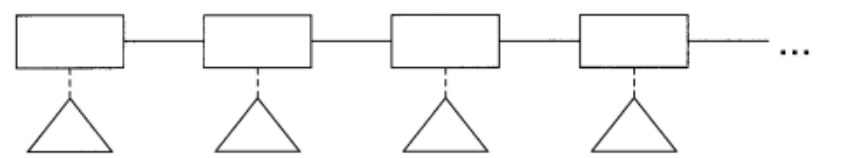(1)if 语句（带有3个可能的孩子）如下所示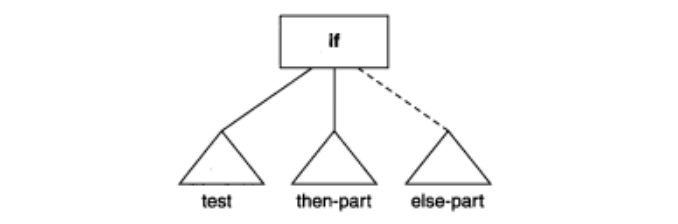(2)repeat 语句有两个孩子。第1个是表示循环体的语句序列，第 2个是一个测试表达式 (3)assign 语句有一个表示其值是被赋予的表达式的孩子（被赋予的变量名保存在语句节点中）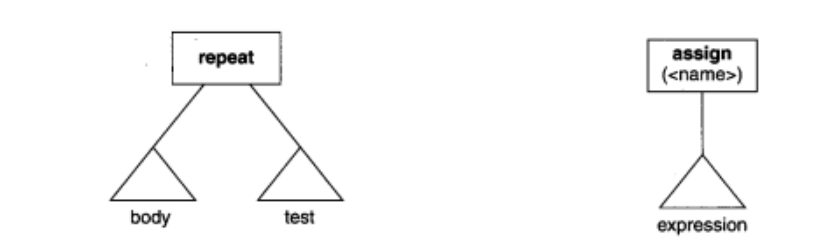(4)write 语句也有一个孩子，它表示要写出值的表达式： (5)算符表达式有两个孩子，它们表示左操作数表达式和右操作数表达式：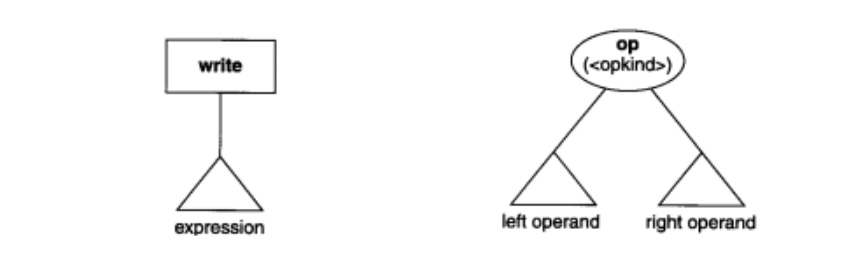其他所有的节点（ r e a d语句、标识符表达式和常量表达式）都是叶子节点。 最后准备显示一个 T I N Y程序的树。程序清单 3 - 3中有来自第1章计算一个整型阶乘的示例程序，它的语法树在图3 - 6中。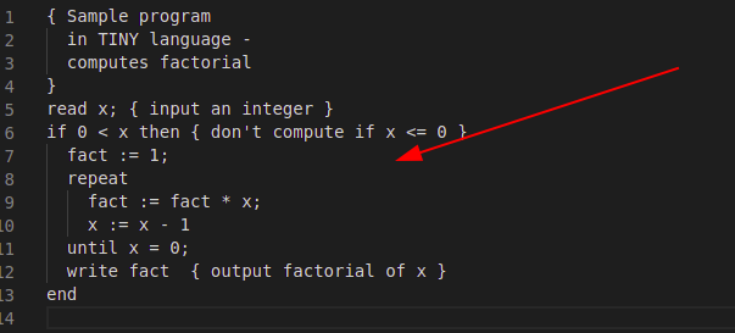我们将会构建出如图所示的语法树：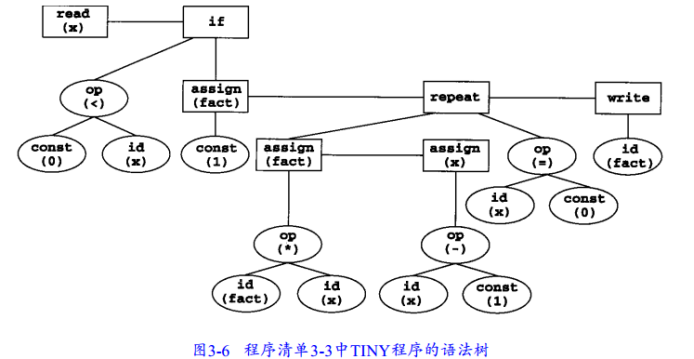第二部分 实现一门语言的语法分析器 实验内容： （1）语言确定： C－语言，其定义在《编译原理及实践》附录A中。也可选择其它语言，不过要有该语言的详细定义（可仿照C－语言）。一旦选定，不能更改，因为要在以后继续实现编译器的其它部分。鼓励自己定义一门语言。也可选择TINY语言，但需要使用与TINY现有语法分析代码不同的分析算法实现，并在实验报告中写清原理。 （2）完成C－语言的BNF文法到EBNF文法的转换： 通过这一转换，消除左递归，提取左公因子，将文法改写为LL（1）文法，以适用于自顶向下的语法分析。规划需要将哪些非终结符写成递归下降函数。 （3）为每一个将要写成递归下降函数的非终结符如：变量声明、函数声明、语句序列、语句、表达式等，定义其抽象语法子树的形式结构，然后定义C－语言的语法树的数据结构。 （4）仿照前面学习的语法分析器，编写选定语言的语法分析器。可以自行选择使用递归下降、LL(0)、LR(0)、SLR、LR(1)中的任意一种方法实现。 （5）准备2~3个测试用例，测试并解释程序的运行结果。 一.语言确定 我在实验3中自定义了一门语言Quary，Quary语言的定义是一个很有挑战性的过程，我模仿C—和python成功定义了它（也许并不完备，随着实验的推进我会一一完善），定义语言的过程中，我对BNF语法有了新的了解和学习。 关于语法和语义，我将仿照C-的定义给出Quary语言的BNF语法： 1.program→declaration-list 2.declaration-list→declaration-listdeclaration|declaration 3.declaration→var-declaration|fun-declaration  程序由声明的列表(或序列)组成，声明可以是函数或变量声明，顺序是任意的。至少必须有一个声明。接下来是语义限制(这些在C中不会出现)。所有的变量和函数在使用前必须声明(这避免了向后backpatching引用)。程序中最后的声明必须是一个函数声明，名字为main。注意，Quary缺乏原型，因此声明和定义之间没有区别 4.var-declaration→type-specifierID;|type-specifierID[NUM]; 5.type-specifier→int|char|void  变量声明或者声明了简单的整数类型int / 字符类型char变量，或者是基类型为整数/字符的数组变量，索引范围从0到 NUM-1。注意，在一个变量声明中，只能使用类型 指示符int/char。void用于函数声明(参见下面)，也要注意，每个声明只能声明一个变量。 6.fun-declaration→type-specifierID(params)compound-stmt 7.params→param-list|void 8.param-list→param-list,param|param 9.param→type-specifierID|type-specifierID[]  函数声明由返回类型指示符、标识符以及在圆括号内的用逗号分开的参数列表组成，后面 跟着一个复合语句，是函数的代码。如果函数的返回类型是void，那么函数不返回任何值(即 是一个过程)。函数的参数可以是void(即没有参数)，或者一列描述函数的参数。参数后面跟 着方括号是数组参数，其大小是可变的。简单的整型参数由值传递。数组参数由引用来传递 (也就是指针)，在调用时必须通过数组变量来匹配。注意，类型“函数”没有参数。一个函数 参数的作用域等于函数声明的复合语句，函数的每次请求都有一个独立的参数集。函数可以是递归的(对于使用声明允许的范围)。 10.compound-stmt→:local-declarationsstatement-list end  复合语句由用: end围起来的一组声明和语句组成。复合语句通过用给定的顺序执行语句 序列来执行。局部声明的作用域等于复合语句的语句列表，并代替任何全局声明。 11.local-declarations→local-declarationsvar-declaration|empty 12.statement-list→statement-liststatement|empty  注意声明和语句列表都可以是空的(非终结符empty表示空字符串，有时写作) 13.statement→expression-stmt 14.|compound-stmt 15.|selection-stmt 16.|iteration-stmt 17.|return-stmt 18.expression-stmt→expression;|;  表达式语句有一个可选的且后面跟着分号的表达式。这样的表达式通常求出它们一方的结 果。因此，这个语句用于赋值和函数调用。 19.selection-stmt→if(expression):statement 20.|if(expression)statement else statement 21.end  if语句有通常的语义：表达式进行计算；非0值引起第一条语句的执行；0值引起第二条语 句的执行，如果它存在的话。这个规则导致了典型的悬挂else二义性，可以用一种标准的方法解决：else部分通常作为当前if的一个子结构立即分析(“最近嵌套”非二义性规则)。 同样的他们用: end包裹起来。 22.iteration-stmt→while(expression): 23.statement 24.end  while语句是Quary中的一种重复语句。它重复执行表达式，并且如果表达式的求值为非0， 则执行语句，当表达式的值为0时结束。 25.iteration-stmt→forEach x in list: 26.statement 27.end  forEach语句是Quary中遍历列表数组的语句，它重复执行表达式，顺序遍历列表直到到达列表尾结束。 28.return-stmt→return;|return expression;  返回语句可以返回一个值也可无值返回。函数没有说明为void就必须返回一个值。函数 声明为void就没有返回值。return引起控制返回调用者(如果它在main中，则程序结束)。 29.expression→var=expression|simple-expression 30.var→ID|ID[expression]  表达式是一个变量引用，后面跟着赋值符号(等号)和一个表达式，或者就是一个简单的表 达式。赋值有通常的存储语义：找到由var表示的变量的地址，然后由赋值符右边的子表达式进行求值，子表达式的值存储到给定的地址。这个值也作为整个表达式的值返回。var是简单的(整型)变量或下标数组变量。负的下标将引起程序停止(与C不同)。然而，不进行下标越界检查。 var表示Quary比C的进一步限制。在C中赋值的目标必须是左值(l-value)，左值是可以由许多操作获得的地址。在Quary中唯一的左值是由var语法给定的，因此这个种类按照句法进行检查，代替像C中那样的类型检查。故在Quary中指针运算是禁止的。 31.simple-expression→additive-expressionrelopadditive-expression|additive-expression 32.relop→<=|<|>|>=|==|!=  简单表达式由无结合的关系操作符组成(即无括号的表达式仅有一个关系操作符)。简单表 达式在它不包含关系操作符时，其值是加法表达式的值，或者如果关系算式求值为ture，其值为1，求值为false时值为0。 33.additive-expression→additive-expressionaddopterm|term 34.addop→+|- 35.term→termmulopfactor|factor 36.mulop→*|/  加法表达式和项表示了算术操作符的结合性和优先级。符号表示整数除；即任何余数都被 截去。 37.26.factor→(expression)|var|call|NUM  因子是围在括号内的表达式；或一个变量，求出其变量的值；或者一个函数调用，求出函 数的返回值；或者一个NUM，其值由扫描器计算。数组变量必须是下标变量，除非表达式由单个ID组成，并且以数组为参数在函数调用中使用(如下所示)。 38.call→ID(args) 39.args→arg-list|empty 40.arg-list→arg-list,expression|expression  函数调用的组成是一个ID(函数名)，后面是用括号围起来的参数。参数或者为空，或者由 逗号分割的表达式列表组成，表示在一次调用期间分配的参数的值。函数在调用之前必须声明，声明中参数的数目必须等于调用中参数的数目。函数声明中的数组参数必须和一个表达式匹配，这个表达式由一个标识符组成表示一个数组变量。最后，上面的规则没有给出输入和输出语句。在C－的定义中必须包含这样的函数，因为 与C不同，C－没有独立的编译和链接工具；因此，考虑两个在全局环境中预定义的函数，好像它们已进行了声明： 41.Int input(void){...} 42.Void print(int x | int x[] |char x | char x[]){...}  input函数没有参数，从标准输入设备(通常是键盘)返回一个整数值。print函数接受 一个整型参数或整型数组或字符参数或字符数组，其值和一个换行符一起打印到标准输出设备(通常是屏幕)。 二.完成BNF到EBNF的转换 递归下降使用拓展巴克斯范式（EBNF）把左递归写成其他形式，类似exp->exp addop term | term这样的表达式是左递归的，并不好直接用递归下降程序来翻译所以通过改写成 exp->term { addop term } 可以方便表示（大括号( { } )内包含的为可重复0至无数次的项。） exp -> exp + num|num #这里就是递归，我们在定义“exp”这个概念，而它的定义里面又用到自己本身！ exp -> term { addop term } #都是匹配形如 num (+ num) (+ num) ... 的串 。在数学上，这两个表达式是等价的。  对应的exp匹配程序如下： def exp(): term(); while token = '+' or token = '-': match(token); term(); end while end  我们只需要将文法定义中的左递归BNF按照规则exp->exp addop term | term改写成 exp->term { addop term } 进行替换即可。 三.定义抽象语法树形式结构 对于抽象语法树的结构，我仿照TINY的语法分析器来定义它。在头文件globals.h中我们定义了一些节点类型的变量和结构，Quary 1.变量和结构 （1）有两种基本的结构类型：语句StmtK和表达式ExpK。 （2）语句共有 5类（ i f语句、 while语句、 assign语句、 Input语句和PrintK语句） （3）表达式共有 3类（算符表达式OpK、常量表达式ConstK和标识符表达式IdK） 。 因此，语法树节点首先按照它是语句还是表达式来分类，接着根据语句或表达式的种类进行再次分类。树节点最大可有 3个孩子的结构（仅在带有 e l s e部分的i f语句中才需要它们） 语句通过同属域而不是使用子域来排序。 节点类型： /*用于分析的语法树*/ typedef enum {StmtK,ExpK} NodeKind; //节点类型  表达式类型： typedef enum {IfK,WhileK,ForEach,AssignK,InputK,PrintK} StmtKind; //语句类（statment）  表达式种类： typedef enum {OpK,ConstK,IdK} ExpKind; //表达式类（expression）算符表达式、常量表达式和标识符表达式  语法树的节点定义： 树的结点种类NodeKind分为StmtK和ExpK两类，两类又有各自的子类。在语法树中标明种类有利于代码生成，当遍历语法树检测到特定类型时就可以进行特定的处理。treeNode结构为指向孩子和兄弟的节点。 语句通过同属域而不是子域来排序，即由父亲到他的孩子的唯一物理连接是到最左孩子的。孩子则在一个标准连接表中自左向右连接到一起，这种连接称作同属连接,用于区别父子连接。 /*ExpType用于类型检查*/ typedef enum {Void,Integer,Boolean} ExpType; //表达式种类 #define MAXCHILDREN 3 //语法树的节点 typedef struct treeNode //语法树的节点 { struct treeNode * child[MAXCHILDREN]; //子节点, 三元 struct treeNode * sibling; //兄弟节点 int lineno; //当前读取到的行号 NodeKind nodekind; //节点类型 //类型 union { StmtKind stmt; //语句类型 ExpKind exp; //表达式类型 } kind; //属性 union { TokenType op; //操作符 int val; //值 char * name; //字段名 } attr; //表达式类型 ExpType type; //表达式类型 } TreeNode;  2.函数功能 （1）在文件parse.c中我们生命并且实现了这样的一些函数，这些函数将会帮助构建我们的语法树： 函数 功能 函数 功能 static TreeNode * stmt_sequence(void); 在循环中匹配分号，再匹配新行（statement） static void match(TokenType expected) 用来判断当前token是否与当前表达式的下一个值匹配 static TreeNode * if_stmt() IF语句规则 static TreeNode * while_stmt(void); 循环语句 static TreeNode * input_stmt(void); Write语句 static TreeNode * simple_exp(void); 匹配加减法表达式 static TreeNode * term(void); 匹配乘除法表达式 static TreeNode * factor(void); 匹配因式项 static TreeNode * forEach_stmt(void); 循环语句规则: forEach static TreeNode * assign_stmt(void); 赋值语句规则: assign static TreeNode * print_stmt(void); 输出语法规则PRINT static TreeNode * express(void); 逻辑表达式 static TreeNode * compareExpress(void); 大小比较表达式 四.仿照语法分析器，编写选定语言的语法分析器 (1)实现思路： 在编写语法分析器时，我选用的方法是递归下降法，基本思路是把图中的文法用程序翻译出来。每个文法都对应一个处理该文法的子例程。每次用stmt_sequence在循环中匹配新的行，然后用match匹配语句开头的第一个字段，判断不同的文法，交给不同的文法处理程序处理。其处理程序的结构关系图如下：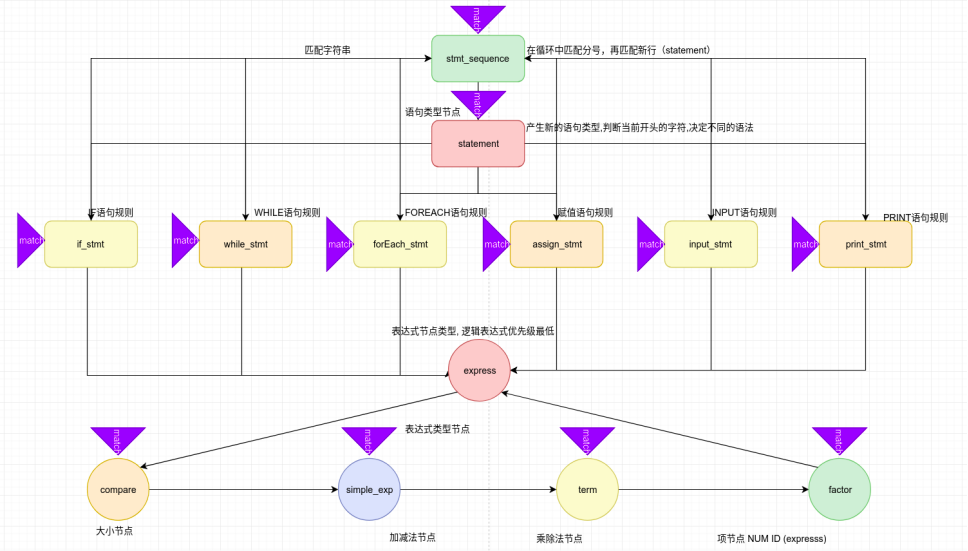经过statement匹配的语句，交给不同的文法处理器处理，包括了if_stmt, while_stmt, forEach_stmt, assign_stmt, input_stmt, print_stmt, 如果匹配错误将会触发异常。在每个语法处理程序中，包含了表达式节点，这个时候语法处理程序将会有express匹配表达式，表达式采用递归的形式构建，按照逻辑顺序，会先后由逻辑表达式节点，大小判断节点，加减法节点，乘除法节点，项节点处理，然后每一个项又可能有新的express组成，这样循环递归执行，直到表达式匹配到表达式类型（expression）算符表达式、常量表达式和标识符表达式{OpK,ConstK,IdK}终止。 (2)下面我会对几个关键的函数做详细介绍： ①stmt_sequence函数在循环中匹配分号，再匹配新的行 //stmt_sequence->stmt_sequence;statement | statement TreeNode * stmt_sequence(void) //在循环中匹配分号，再匹配新行（statement） { TreeNode * t = statement(); TreeNode * p = t; // if(token==SEMI) match(SEMI); // if(token==ENDFILE) match(ENDFILE); // if(token==SEMI) match(SEMI); //当遇到文件结束, END保留字的时候单独一行 while ((token!=ENDFILE) && (token!=END) &&(token!=ELSE)) { TreeNode * q; //匹配当前是不是; 或 :号, 这个时候表示新的一行结束 // if(token==SEMI) match(SEMI); // else // match(COLON); q = statement(); //增加兄弟节点 if (q!=NULL) { if (t==NULL) t = p = q; else /* now p cannot be NULL either */ { p->sibling = q; p = q; } } } return t; }  ② token是一个静态全局变量这个是主循环。在循环中匹配分号，再匹配新行（statement） TreeNode * stmt_sequence(void) { TreeNode * t = statement(); TreeNode * p = t; while ((token!=ENDFILE) && (token!=END) && (token!=ELSE) && (token!=UNTIL)) { TreeNode * q; match(SEMI); q = statement(); if (q!=NULL) { if (t==NULL) t = p = q; else /* now p cannot be NULL either */ { p->sibling = q; p = q; } } } return t; }  ③ 然后在statement函数中根据首token的类别通过switch case将解析交给match函数：用来判断当前token是否与当前表达式的下一个值匹配 /* statement → expression-stmt | statement */ //产生新的语句类型,判断当前开头的字符,决定不同的语法 TreeNode * statement(void) { TreeNode * t = NULL; switch (token) { case IF : t = if_stmt(); break; //IF case WHILE : t = while_stmt(); break; //WHILE case FOREACH: t=forEach_stmt(); break; //FOREACH case ID : t = assign_stmt(); break; //赋值 case INPUT: t = input_stmt(); break; //输入 case PRINT: t = print_stmt(); break; //输出 // case SEMI: match(SEMI);break; default : syntaxError("unexpected token -> "); //其他状态认为是错误状态 printToken(token,tokenString); token = getToken(); break; } return t; }  ④ 对if语句来说，if_stmt : IF exp THEN stmt_seq END 在句法的严格限制下，if，then，end三个关键字是必须匹配的，match进行“匹配”确认，错误就报错，成功则运行词法分析读取下一个token TreeNode * if_stmt(void) { TreeNode * t = newStmtNode(IfK); match(IF); if (t!=NULL) t->child = exp(); match(THEN); if (t!=NULL) t->child = stmt_sequence(); if (token==ELSE) { match(ELSE); if (t!=NULL) t->child = stmt_sequence(); } match(END); return t; }  ⑤对于WHILE循环语句，他总是先匹配保留字WHILE，然后再匹配接着他的”:”， 然后匹配执行语句，他只有一个子节点，递归使用stmt_sequenc(), 最后匹配终结符号end。 //循环语句规则: WHILE TreeNode * while_stmt(void) { TreeNode * t = newStmtNode(WhileK); //产生一个新的语句WHILE点 match(WHILE); //匹配WHILE if (t!=NULL) t->child = express(); //匹配紧跟着的条件表达式 match(COLON); //匹配: if (t!=NULL) t->child = stmt_sequence(); //匹配执行语句 match(END); //匹配一个END return t; }  ⑥对于赋值语句语句，他总是先设置属性name为当前的串，然后匹配一个新的ID和=号，最后通过express匹配子节点表达式。 //赋值语句规则: assign TreeNode * assign_stmt(void) { TreeNode * t = newStmtNode(AssignK); if ((t!=NULL) && (token==ID)) //name属性 t->attr.name = copyString(tokenString); match(ID); //匹配一个ID match(ASSIGN); //匹配一个= if (t!=NULL) t->child = express(); //匹配表达式 return t; }  ⑦ 输入输出语法类似，首先匹配保留字，然后添加name属性，对于PRINT，子节点点则是一个表达式。 //输入语法规则INPUT TreeNode * input_stmt(void) { TreeNode * t = newStmtNode(InputK); match(INPUT); //匹配INPUT保留字 if ((t!=NULL) && (token==ID)) t->attr.name = copyString(tokenString); //添加属性 match(ID); return t; } //输出语法规则PRINT TreeNode * print_stmt(void) { TreeNode * t = newStmtNode(PrintK); match(PRINT); //匹配输出PRINT if (t!=NULL) t->child = express(); //添加子节点为表达式 return t; }  ⑦下面是表达式的处理，表达式处理具有高度的一致性，表达式采用递归的形式构建，按照逻辑顺序，会先后由逻辑表达式节点，大小判断节点，加减法节点，乘除法节点，项节点处理，然后每一个项又可能有新的express组成，这样循环递归执行，直到表达式匹配到表达式类型（expression）算符表达式、常量表达式和标识符表达式{OpK,ConstK,IdK}终止。如下图：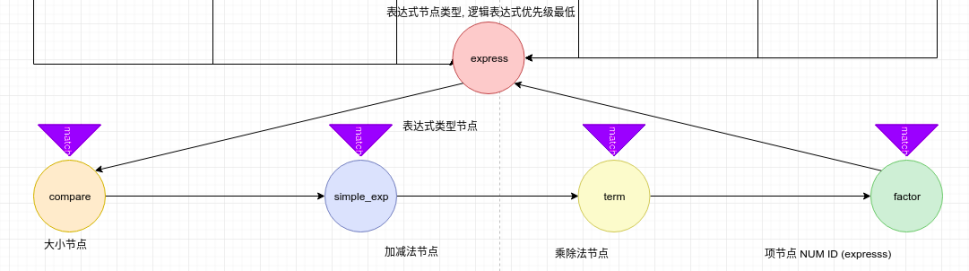//大小比较表达式 //var = compareExpress | simple_exp TreeNode * compareExpress(void) { TreeNode * t = simple_exp(); if ((token==LS)||(token==LE)||(token==GT)||(token==GE)||(token==EQ)||(token==NE)){ TreeNode * p = newExpNode(OpK); //新建一个表达式节点, 类型为算符表达式 if (p!=NULL) { p->child = t; // p->attr.op = token; //操作符为token t = p; // } match(token); //匹配当前字符防止检测发生 if (t!=NULL) t->child = simple_exp(); //子表达式 } return t; }  上面是一大小表达式的例子，剩下的大小表达式compareExpress，加减法表达式simple_exp，乘除法表达式term与此类似。 ⑧项的处理： 一个表达式expresss可能由子表达式， ID或NUM组成，也就是ENBF表达式(expression) | NUM | ID ，因此我们可以这样处理项，判断当前的串如果是一个NUM，那么新建一个子节点，将它的val设置为当前串的值; 如果当前串是ID，那么新建节点设置属性为当前串; 如果当前串是( 那么要匹配子表达式调用函数express，然后匹配)号。 TreeNode * factor(void) { TreeNode * t = NULL; switch (token) { case NUM : //NUM类型表达式 t = newExpNode(ConstK); if ((t!=NULL) && (token==NUM)) t->attr.val = atoi(tokenString); match(NUM); break; case ID : //ID标识符类型表达式 t = newExpNode(IdK); if ((t!=NULL) && (token==ID)) t->attr.name = copyString(tokenString); match(ID); break; case LPAREN : //左括号表达式 match(LPAREN); t = express(); match(RPAREN); //匹配右括号 break; // case SEMI: match(SEMI);break; default: syntaxError("unexpected token -> "); printToken(token,tokenString); token = getToken(); break; } return t; }  经过上面的处理，语法树就能成功构建起来，为了输出语法树，我们还需要借助工具类中的函数printTree将节点打印出来，基本思路很简单，对于当前的一个节点，我们要先判断他的类型，如果是语句类型，直接打印;如果是表达式类型，那么打印属性。然后扩展所有的子节点。 void printTree( TreeNode * tree ) { int i; INDENT; while (tree != NULL) { printSpaces(); if (tree->nodekind==StmtK) //如果是语句类型 { switch (tree->kind.stmt) { case IfK,WhileK,ForEach,AssignK,InputK,PrintK: } } else if (tree->nodekind==ExpK) //如果是表达式类型 { switch (tree->kind.exp) { case OpK: printToken(tree->attr.op,"\0"); case ConstK: fprintf(listing,"Const: %d\n",tree->attr.val); case IdK: fprintf(listing,"Id: %s\n",tree->attr.name); } } //扩展兄弟节点 for (i=0;i<MAXCHILDREN;i++) //兄弟节点 printTree(tree->child[i]); tree = tree->sibling; } UNINDENT; }  五.样例测试 我共设置了三组样例，下面我将会对他们一一分析: （1）样例1： 下图左侧样例1是一个简单的if条件语句测试，首先使用input输入一个值x，然后用对x进行判断，如果x小于0,那么facts=0；如果x> 2, 那么facts=1, 如果x > 3, 那么facts=2， 否则facts=3。最后将facts用print打印出来。 执行命令：$ ./QuaryParse samples/sample1.py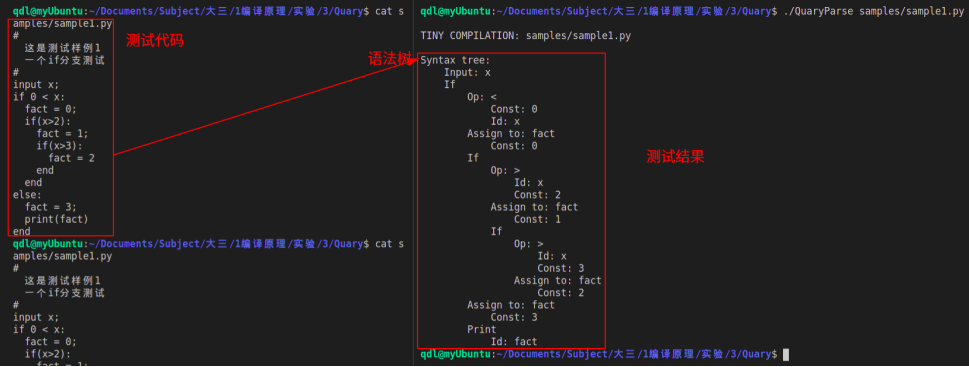上图右侧是样例1的执行结果，首先语法树的第一层应该有两个节点，也就是input和IF，然后IF语句内部则有两个分支，一个是从fact=0到end，另外一个是else部分，在地一个分支内部存在一个IF语句，同样的，IF语句内部又有一个IF语句嵌套，因此有三层IF，然后处理Else分支，在Else分支中只有两个语句，一个是赋值语句fact=3,相应的语法树结果为Assign to: fact，Const: 3 最后是Print语句，内部是一个表达式ID ：fact，观察测试结果，符合预期。
（2）样例2：
下图左侧样例2是一个while-if-else分支测试，程序求和0~10的平方和,输入一个值,判断sum和他的大小关系，下面是测试结果：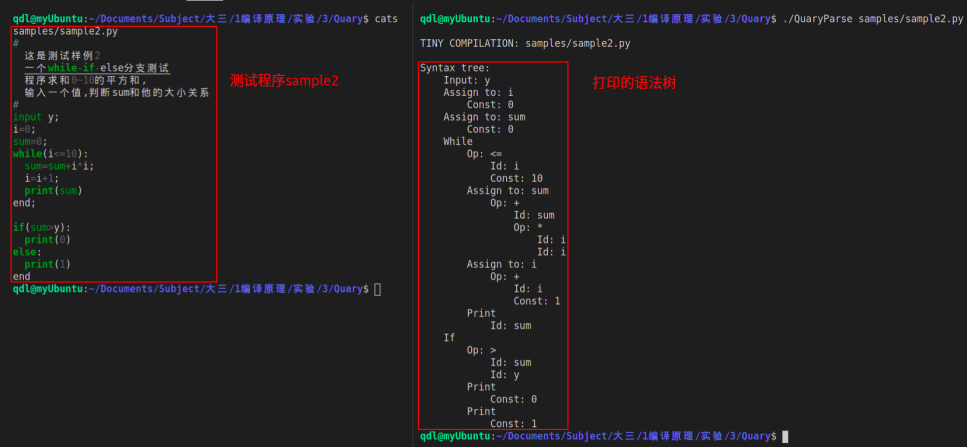观察测试代码，语法树第一层有4个语句节点，分别是Input，Assign，Assign，While，If，然后我们看到重点位置while循环，在while循环内部有3条语句，因此有三个语句节点，他们分别是赋值语句Assign，Assign，打印语句Print，在while语句的后面，我们还有一个表达式节点，他用于确定循环执行的条件，Op: <= \ Id: i \ Const: 10，最后IF语句有两个分支，后面紧跟一个表达式节点Op: > \ Id: sum \ Id: y ，接下来就是else分支，Print \ Const: 1,观察测试结果，符合分析预期。
（3）样例3：
sample3.qy 测试样3, 它计算一个斐波拉契数列Fibonacci的值，输入一个数x，程序计算Fibonacci(x) 并且打印，下面我将仔细分析语法树的结果。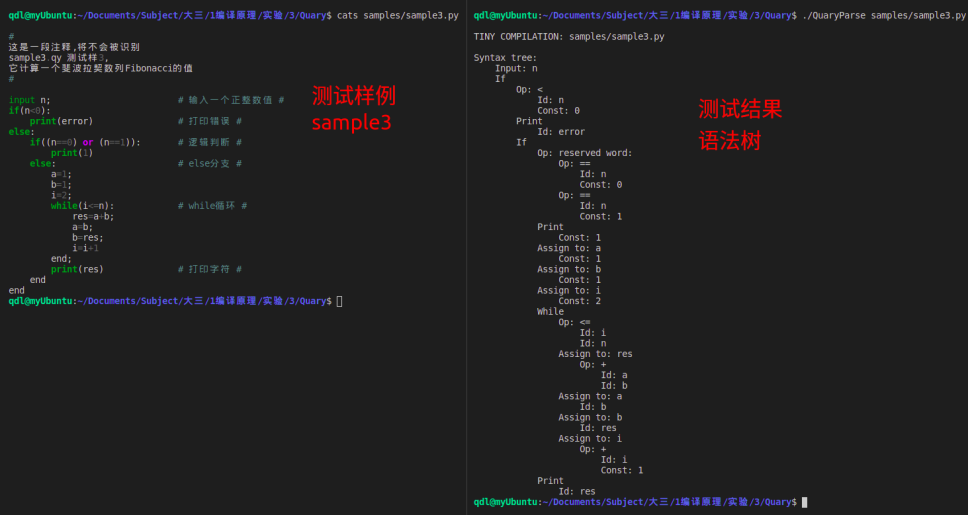观察测试代码，语法树第一层有2个语句节点，分别是Input，If。第一个节点Input: n输入n的值，然后来到IF节点，他只是简单判断n<0 , 关键部分还在ELSE分支，在分支中，有一个逻辑表达式，if((n0) or (n1)):，程序将条件识别为两个表达式，Op: == \ Id: n \ Const: 0 然后两个express用保留子OR组合成一个新的表达式节点。在IF的子分支ELSE中，是一连串的数据赋值操作，毫无疑问他们被处理为Assign to:  \ const，最后是一段while循环，首先生成表达式节点express，然后是一段连续的赋值交换操作和表达式计算                Assign to: res \ Op: + Id: a \ Id: b, 观察测试结果，符合分析预期。


展开全文• 实验要求 【任务介绍】根据给定源语言的构词规则，从任意字符串中识别出该语言所有的合法的单词符号，并以等长的二元组形式输出。 【输入】字符串形式的源程序。 【输出】单词符号所构成的串（流），单词以等长的...
实验要求
【任务介绍】根据给定源语言的构词规则，从任意字符串中识别出该语言所有的合法的单词符号，并以等长的二元组形式输出。
【输入】字符串形式的源程序。
【输出】单词符号所构成的串（流），单词以等长的二元组形式呈现。
【题目】设计一个程序，根据给定源语言的构词规则，从任意字符串中识别出该语言所有的合法的单词符号，并以等长的二元组形式输出。注意：

该程序应该设计为至少包含2个模块：驱动模块和工作模块。驱动模块包含了程序的入口和出口，主要负责输入、输出处理并调用工作模块；工作模块负责具体的分割、识别、归类等工作。这样做的好处是：只要模块间的接口（需要传递哪些数据，数据的结构）设计合理，后续实验中做语法分析器时就可以直接调用此处的工作模块，而不需要改动太多代码。

编程环境和语言
编程语言：C++
IDE：vs 2019
实验原理分析
词法分析中，实现的功能是分割每个单词，同时判断每个单词的类型，并二元组的形式将其输出。首先需要预处理将注释和多余的空白字符去除，之后依次读取单词，对其进行判断，然后根据其类型输出其特定的二元组形式。
程序关键部分分析
用来预处理的函数为pre_process(char *buff, int &in_comment)（和实验二一致），用来将程序中的单词区分提取出来的函数为scanner(ifstream& fin, ofstream& fout)。
定义
string token;  //用于暂存单词序列
string ID[MAXN];  //用于存储程序中的所有标识符
int IDNum = 0;  //用于记录标识符的数量
int digitNum = 0;  //用于记录常数的数量

char keywords = {  //关键字,包括main在内共有33个
"auto", "short", "int", "long", "float", "double", "char", "struct",
"union", "enum", "typedef", "const", "unsigned", "signed", "extern",
"register", "static", "volatile", "void", "if", "else", "switch",
"case", "for", "do", "while", "goto", "continue", "break", "default",
"sizeof", "return", "main"
};
char operators = {  //运算符,共38个
"+", "-", "*", "/", "%", "++", "--", "==", "!=", ">", ">=", "<", "<=",
"&&", "||", "!", "=", "+=", "-=", "*=", "/=", "%=", "<<=", ">>=", "&=",
"^=", "|=", "&", "|", "^", "~", "<<", ">>", "?", ":", ",", ".", "->"
};
char delimiters = { '(', ')', '[', ']', '{', '}' , ';'};  //分隔符,共7个

函数定义为：
bool isKey(char* s);  //用来判断字符串是否为关键字,是则返回true,否则返回false
bool isOP(char* s);  //用来判断字符串是否为运算符,是则返回true,否则返回false
bool isDE(char s);  //用来判断字符是否为分隔符,是则返回true,否则返回false
int findID(const string &s);  //找出标识符在ID中的位置,找到返回数组的下标,否则返回-1
void pre_process(char *buff, int &in_comment);  //预处理
void scanner(ifstream& fin, ofstream& fout);

关键部分分析
这里就不再详细介绍pre_process()函数的关键部分了（详情可以参考《实验二：标识符的识别》），着重介绍scanner()函数：
这里我实现的结果可以表示为：
(标识符, location)  //location表示标识符第一次出现时在标识符中的位置,同一标识符的位置具有唯一性
(关键字, _)
(运算符, _)
(界符, _)
(常数, times)  //times表示常数出现的次数,这里若第一次和第二次出现的常数都是1,则输出为(1, 1)和(1, 2),即默认所有常数都不相等
(字符串常量, _)

同时，若常数长度为1，则直接输出，若长度大于1，且第一个字符为0，则直接报错，不输出该常数；对于标识符，若标识符长度大于15，则报错，不输出该标识符。
while (i < strlen(buff)) {
if (buff[i] == ' ' && j > 0) {  //遇到空格,则说明上一个词已经结束了
words[j] = '\0';
token = words;
if (isOP(words) || isKey(words) || isDE(words)) {  //若是运算符、关键字和界符,处理方法一样
//cout << "(" << token << ", _)" << endl;
if (!first) fout << '\n';
else first = false;
fout<< "(" << token << ", _)";
}
else if (isdigit(words)) {  //若是常数
if (j == 1) {  //若常数的位数为1,则不用判断第一个是否为0
//cout << "(" << token << ", " << 1 + digitNum++ << ")" << endl;
if (!first) fout << '\n';
else first = false;
fout << "(" << token << ", " << 1 + digitNum++ << ")";
}
else {
if (words == '0') {
//cout << "(" << token << ", " << 1 + digitNum++ << ")" << endl;
if (!first) fout << '\n';
else first = false;
fout << "(" << token << ", " << 1 + digitNum++ << ")";
}
else {  //常数的第一个字符不能为0
cout << "ERROR: The first digit cannot be 0!" << endl;
}
}
}
else if (words == '\''||words == '\"') {  //若是字符串常量
//cout << "(" << token << ", _)" << endl;
if (!first) fout << '\n';
else first = false;
fout<< "(" << token << ", _)";
}
else {  //否则为标识符
if (token.length() <= 15) {  //标识符最长为15个字符
int location = findID(token);  //首先判断该标识符是否已经存在了
if (location == -1) {  //若没有找到,则将该标识符存入ID数组中,并且可以知道该标识符所在的位置就是IDNum的值
ID[IDNum++] = token;
//cout << "(" << token << ", " << IDNum << ")" << endl;
if (!first) fout << '\n';
else first = false;
fout<< "(" << token << ", " << IDNum << ")";
}
else {
//cout << "(" << token << ", " << location + 1 << ")" << endl;
if (!first) fout << '\n';
else first = false;
fout<< "(" << token << ", " << location + 1 << ")";
}
}
else {
cout << "ERROR Identifier length can't exceed 15 characters" << endl;
}
}
j = 0;
}
else {
words[j++] = buff[i];
}
i++;
}

程序测试
测试用例：
int main(){
int a;
int b;

a = 1;
b = a + 2 ;

return b;
}

输出结果为：
(int, _)
(main, _)
((, _)
(), _)
({, _)
(int, _)
(a, 1)
(;, _)
(int, _)
(b, 2)
(;, _)
(a, 1)
(=, _)
(1, 1)
(;, _)
(b, 2)
(=, _)
(a, 1)
(+, _)
(2, 2)
(;, _)
(return, _)
(b, 2)
(;, _)
(}, _)

总结
首先可以看到，这里我只是用了一个token暂时存放单词，然后用一个ID[]数组来确保标识符的唯一性，并没有用到符号表。
龙书（编译原理之神）上是说词法分析中需要用到符号表将不同类型的单词区分并存储，但是我们要考虑到一个事实，即至少目前为止，符号表并没有什么用处。符号表真正的用途体现在语义分析中，即判断标识符的作用域时需要用到符号表，而符号表在词法分析和语法分析中可有可无，所以这里我们采用的是软件工程中的一个概念：迭代开发。因为暂时用不到，所以暂时没必要考虑太多，否则问题会越来越复杂！
完整代码
#include<iostream>
#include<fstream>
#include<string>
#include<cctype>
#define MAXN 1024  //缓冲区的最大容量
using namespace std;

string token;  //用于暂存单词序列
string ID[MAXN];  //用于存储程序中的所有标识符
int IDNum = 0;  //用于记录标识符的数量
int digitNum = 0;  //用于记录常数的数量

char keywords = {  //关键字,包括main在内共有33个
"auto", "short", "int", "long", "float", "double", "char", "struct",
"union", "enum", "typedef", "const", "unsigned", "signed", "extern",
"register", "static", "volatile", "void", "if", "else", "switch",
"case", "for", "do", "while", "goto", "continue", "break", "default",
"sizeof", "return", "main"
};
char operators = {  //运算符,共38个
"+", "-", "*", "/", "%", "++", "--", "==", "!=", ">", ">=", "<", "<=",
"&&", "||", "!", "=", "+=", "-=", "*=", "/=", "%=", "<<=", ">>=", "&=",
"^=", "|=", "&", "|", "^", "~", "<<", ">>", "?", ":", ",", ".", "->"
};
char delimiters = { '(', ')', '[', ']', '{', '}' , ';'};  //分隔符,共7个

bool isKey(char* s) {  //用来判断字符串是否为关键字,是则返回true,否则返回false
for (int i = 0; i < sizeof(keywords) / sizeof(keywords); i++) {
if (strcmp(s, keywords[i]) == 0) return true;
}return false;
}

bool isOP(char* s) {  //用来判断字符串是否为运算符,是则返回true,否则返回false
for (int i = 0; i < sizeof(operators) / sizeof(operators); i++) {
if (strcmp(s, operators[i]) == 0) return true;
}return false;
}

bool isDE(char &s) {  //用来判断字符是否为分隔符,是则返回true,否则返回false
if (strchr(delimiters, s) != NULL) return true;
return false;
}

int findID(const string &s) {  //找出标识符在ID中的位置,找到返回数组的下标,否则返回-1
int i = 0;
while (i < IDNum) {
if (ID[i] == s) return i;
i++;
}return -1;
}

void pre_process(char* buff, int& in_comment) {  //预处理
char data[MAXN] = { '\0' };  //用来存储处理过的数据
char old_c = '\0';  //用来存储上一个字符
char cur_c;  //用来存储当前字符
int i = 0;  //计数器,记录buff
int j = 0;  //计数器，记录data
while (i < strlen(buff)) {  //去注释
cur_c = buff[i++];  //首先将获取的字符存入缓存中
switch (in_comment) {
case 0:
if (cur_c == '\"') {  //进入双引号中
data[j++] = cur_c;
in_comment = 3;
}
else if (cur_c == '\'') {  //进入单引号中
data[j++] = cur_c;
in_comment = 4;
}
else if (old_c == '/' && cur_c == '*') {  //进入多行注释中
j--;
in_comment = 1;
}
else if (old_c == '/' && cur_c == '/') {  //进入单行注释中
j--;
in_comment = 2;
}
else {  //其他情况则直接将数据写入data中
data[j++] = cur_c;
}
break;
case 1:if (old_c == '*' && cur_c == '/') in_comment = 0;  //多行注释结束
break;
case 2:if (i == strlen(buff)) in_comment = 0;  //单行注释到这行结束时标志位置为0
break;
case 3:
data[j++] = cur_c;
if (cur_c == '\"') in_comment = 0;
break;
case 4:
data[j++] = cur_c;
if (cur_c == '\'') in_comment = 0;
break;
}
old_c = cur_c;  //保留上一个字符
}

i = 0;
int k = 0;
while (k < j) {  //分隔词
if (isalpha(data[k]) || data[k] == '_') {  //若为字母或_
while (!isDE(data[k]) && strchr("+-*/%=^~&|!><?:,.", data[k]) == NULL && !isspace(data[k])) {
buff[i++] = data[k++];
}buff[i++] = ' ';
}
else if (isdigit(data[k])) {  //若为数字
while (isdigit(data[k])) {
buff[i++] = data[k++];
}buff[i++] = ' ';
}
else if (isspace(data[k])) {
while (isspace(data[k])) {  //若为空白字符
k++;
}
}
else if (isDE(data[k])) {  //若为界符
buff[i++] = data[k++];
buff[i++] = ' ';
}
else if (data[k] == '\"') {  //若为双引号
buff[i++] = data[k++];
while (data[k] != '\"')  buff[i++] = data[k++];
buff[i++] = data[k++];
buff[i++] = ' ';
}
else if (data[k] == '\'') {  //若为单引号
buff[i++] = data[k++];
while (data[k] != '\'')  buff[i++] = data[k++];
buff[i++] = data[k++];
buff[i++] = ' ';
}
else if (strchr("+-*/%=^~&|!><?:,.", data[k]) != NULL) {  //若为运算符,再查看下一个字符,要尽可能多包含一些运算符
switch (data[k]) {
case '+':buff[i++] = data[k++];
if (data[k] == '+' || data[k] == '=') buff[i++] = data[k++];  //为++或+=运算符
break;
case '-':buff[i++] = data[k++];
if (data[k] == '-' || data[k] == '=' || data[k] == '>') buff[i++] = data[k++];  //为--或-=或->运算符
break;
case '*':buff[i++] = data[k++];
if (data[k] == '=') buff[i++] = data[k++];  //为*=运算符
break;
case '/':buff[i++] = data[k++];
if (data[k] == '=') buff[i++] = data[k++];  //为/=运算符
break;
case '%':buff[i++] = data[k++];
if (data[k] == '=') buff[i++] = data[k++];  //为%=运算符
break;
case '=':buff[i++] = data[k++];
if (data[k] == '=') buff[i++] = data[k++];  //为==运算符
break;
case '^':buff[i++] = data[k++];
if (data[k] == '=') buff[i++] = data[k++];  //为^=运算符
break;
case '&':buff[i++] = data[k++];
if (data[k] == '&' || data[k] == '=') buff[i++] = data[k++];  //为&&或&=运算符
break;
case '|':buff[i++] = data[k++];
if (data[k] == '|' || data[k] == '=') buff[i++] = data[k++];  //为||或|=运算符
break;
case '!':buff[i++] = data[k++];
if (data[k] == '=') buff[i++] = data[k++];  //为!=运算符
break;
case '>':buff[i++] = data[k++];
if (data[k] == '=') buff[i++] = data[k++];  //为>=运算符
else if (data[k] == '>') {
buff[i++] = data[k++];  //为>>运算符
if (data[k] == '=') buff[i++] = data[k++];  //为>>=运算符
}break;
case '<':buff[i++] = data[k++];
if (data[k] == '=') buff[i++] = data[k++];  //为<=运算符
else if (data[k] == '<') {
buff[i++] = data[k++];  //为<<运算符
if (data[k] == '<') buff[i++] = data[k++];  //为<<=运算符
}break;
default:buff[i++] = data[k++];
}buff[i++] = ' ';
}
}
if (i > 0) buff[i] = '\0';  //处理完以后,会在最后留上一个空格
else buff[i] = '\0';
}

void scanner(ifstream& fin, ofstream& fout) {
char buff[MAXN] = { '\0' };  //用来存储读入的数据
char words[MAXN] = { '\0' };  //用来存储词
bool first = true;  //用来判断是否为第一个词
int in_comment = 0;  //0表示当前字符未在注释里,1表示在多行注释中,2表示在单行注释中,3表示在双引号中,4表示在单引号中
char cur_c;  //用来存储当前字符
int i = 0;  //计数器,记录buff
int j = 0;  //计数器,用来记录每个词的长度
while (fin.getline(buff, sizeof(buff))) {
pre_process(buff, in_comment);  //首先预处理,去掉注释,词与词之间、词与运算符之间用一个空格隔开

while (i < strlen(buff)) {
if (buff[i] == ' ' && j > 0) {  //遇到空格,则说明上一个词已经结束了
words[j] = '\0';
token = words;
if (isOP(words) || isKey(words) || isDE(words)) {  //若是运算符、关键字和界符,处理方法一样
//cout << "(" << token << ", _)" << endl;
if (!first) fout << '\n';
else first = false;
fout<< "(" << token << ", _)";
}
else if (isdigit(words)) {  //若是常数
if (j == 1) {  //若常数的位数为1,则不用判断第一个是否为0
//cout << "(" << token << ", " << 1 + digitNum++ << ")" << endl;
if (!first) fout << '\n';
else first = false;
fout << "(" << token << ", " << 1 + digitNum++ << ")";
}
else {
if (words == '0') {
//cout << "(" << token << ", " << 1 + digitNum++ << ")" << endl;
if (!first) fout << '\n';
else first = false;
fout << "(" << token << ", " << 1 + digitNum++ << ")";
}
else {  //常数的第一个字符不能为0
cout << "ERROR: The first digit cannot be 0!" << endl;
}
}
}
else if (words == '\''||words == '\"') {  //若是字符串常量
//cout << "(" << token << ", _)" << endl;
if (!first) fout << '\n';
else first = false;
fout<< "(" << token << ", _)";
}
else {  //否则为标识符
if (token.length() <= 15) {  //标识符最长为15个字符
int location = findID(token);  //首先判断该标识符是否已经存在了
if (location == -1) {  //若没有找到,则将该标识符存入ID数组中,并且可以知道该标识符所在的位置就是IDNum的值
ID[IDNum++] = token;
//cout << "(" << token << ", " << IDNum << ")" << endl;
if (!first) fout << '\n';
else first = false;
fout<< "(" << token << ", " << IDNum << ")";
}
else {
//cout << "(" << token << ", " << location + 1 << ")" << endl;
if (!first) fout << '\n';
else first = false;
fout<< "(" << token << ", " << location + 1 << ")";
}
}
else {
cout << "ERROR Identifier length can't exceed 15 characters" << endl;
}
}
j = 0;
}
else {
words[j++] = buff[i];
}
i++;
}
i = j = 0;
}
if (in_comment != 0) fout << '\n' << "error";  //若标志位不等于0,则说明多行注释不到位,没有结束标志
}

int main() {
ifstream fin("input.txt");
if (!fin.is_open()) cout << "文件不存在!";
ofstream fout("output.txt");
scanner(fin, fout);

fin.close(); fout.close();
return 0;
}



展开全文• 参考C语言版本，用Java写的递归下降分析程序，能对词法分析程序所提供的单词序列进行语法检查和结构分析。被分析的语言应该是PL/0，语法表示如下： (1)<程序>：：＝begin<语句串>end (2)<语句串>：：＝<语句>{；...
• 通过上机实习，加深对语法制时翻译原理的理解，掌握将语法分析所识别的语法成分变换为中间代码的语义翻译方法。 采用递归下降语法制导翻译法对算术表达式、赋值语句、条件语句、循环语句进行语义分析生成四元式序列...
• PS：本次语法分析器实验在我的实验一词法分析器的基础上完成，我把实验一的词法器类稍作修改获得代码的token串以支持语法分析器类LL1，参考了其他博主的first follow集求解代码以及预测分析表的生成代码，注意要修改...c++
• 在实现词法、语法分析程序的基础上，编写相应的语义子程序，进行语义处理，加深对语法制导翻译原理的理解，进一步掌握将语法分析所识别的语法范畴变换为某种中间代码（四元式）的语义分析方法，并完成相关语义分析器......